# Angles

The triangle is one outer angle 158°54' and one internal angle 148°. Calculate the other internal angles of a triangle.

Result

A =  21.1 °
B =  10.9 °

#### Solution:

$A = 180 - 158.9 = 21.1 = \dfrac{ 211 }{ 10 } = 21.1 ^\circ = 21^\circ 6'$
$C = 148 \ ^\circ \ \\ B = 180 - (A+C) = 180 - (21.1+148) = \dfrac{ 109 }{ 10 } = 10.9 = 10.9 ^\circ = 10^\circ 54'$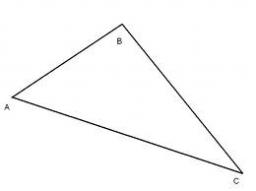Our examples were largely sent or created by pupils and students themselves. Therefore, we would be pleased if you could send us any errors you found, spelling mistakes, or rephasing the example. Thank you!

Leave us a comment of this math problem and its solution (i.e. if it is still somewhat unclear...):Be the first to comment!Tips to related online calculators

## Next similar math problems: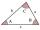The size of two internal angles of a triangle ABC are α=6/18π and β=7/18π. Calculate the size of the third angle.
2. Angles in triangle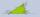Calculate the alpha angle in the triangle if beta is 61 degrees and 98 gamma degrees.
3. Six-sided polygon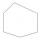In a six-sided polygon. The first two angles are equal, the third angle is twice (the equal angles), two other angles are trice the equal angle, while the last angle is a right angle. Find the value of each angle.
4. TrianglesFind out whether given sizes of the angles can be interior angles of a triangle: a) 23°10',84°30',72°20' b) 90°,41°33',48°37' c) 14°51',90°,75°49' d) 58°58',59°59',60°3'
5. AnglesIn the triangle ABC, the ratio of angles is: a:b = 4: 5. The angle c is 36°. How big are the angles a, b?
6. Triangle P2Can triangle have two right angles?
7. Angles 1It is true neighboring angles have not common arm?
8. 3-bracket 2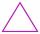May be the smallest angle in the triangle greater than 40°?
9. Isosceles triangleThe circumference of the isosceles triangle is 32.5 dm. Base length is 153 cm. How long is the leg of this triangle?
10. 3-bracketMay be the largest angle in the triangle less than 20°?
11. Arm-legCalculate the length of the base of an isosceles triangle with a circumference 224 cm if the arm length is 68 cm.
12. 7 triangle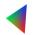The triangle area is 26.7 cm2. Determine the side length l if appropriate height hl = 45.3 cm.
13. Isosceles trapezium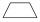Trapezoid YSED (YS||ED) is isosceles. The size of the angle at vertex Y is 17 degrees. Calculate the size of the angle at vertex E.
14. HotelThe hotel has a p floors each floor has i rooms from which the third are single and the others are double. Represents the number of beds in hotel.
15. ExpressionSolve for a specified variable: P=a+4b+3c, for a
16. SimplifySimplify the following problem and express as a decimal: 5.68-[5-(2.69+5.65-3.89) /0.5]
17. AlleyAlley measured a meters. At the beginning and end are planted poplar. How many we must plant poplars to get the distance between the poplars 15 meters?